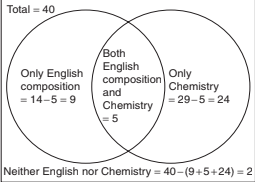# Mathematical Aptitude - Venn Diagram

>>>>>>>>Venn Diagram

• A

2• B

3• C

4• D

5• Option : A
• Explanation :Alternatively: Students taking either English composition or Chemistry = 14 + 29 – 5 = 38
Hence, students not taking any class = 40 − 38 = 2

• A

83%• B

23%• C

64%• D

55.5%• Option : B
• Explanation : Number of candidates failed in English = 52%
Number of candidates failed in Mathematics = 42%
Number of candidates failed in both = 17%
Total failed = 52 + 42 – 17 = 77%
Passed in both the subjects = 100 − 77 = 23%

• A

10%• B

15%• C

20%• D

25%• Option : C
• Explanation : People studying newspaper X or Y = 40 + 50 − 10 = 80.
Hence, people studying neither X nor Y = 100 − 80 = 20.
(Candidates can try to solve this question with Venn diagram also.)

Study the diagram given below and answer each of the following questions.• A

17• B

5• C

10• D

30Study the diagram given below and answer each of the following questions.• A

22• B

8• C

3• D

30## Description

• Venn Diagrams Questions can be used to give quizzes by any candidate who is preparing for UGC NET Paper 1
• This Venn Diagrams Questions section will help you test your analytical skills in a tricky method, thereby giving you an edge over other students
• All candidates who have to appear for the Kendriya Vidyalaya Entrance exam can also refer to this mcq section.
• You can also get access to the Venn Diagrams MCQ ebook.
• Venn Diagrams Questions can be used in the preparation of JRF, CSIR, and various other exams.
• You can also download pdf for these Venn Diagrams multiple-choice questions Answers.
• This Venn Diagrams Multiple Choice Questions Answers section can also be used for the preparation of various competitive exams like UGC NET, GATE, PSU, IES, and many more.
• Venn Diagrams Questions can be used to gain a credit score in various undergraduate and postgraduate courses like Bcom, Mcom and more

Related Quiz.
Venn Diagram